# The Acid Test - More Useful Calculations for HPLC Eluent Preparation

It would seem that my most recent Tech Tips are of a rather mathematical slant. I’m not sure why as I base my musings on inspiration from everyday life in our laboratory and those of our clients. Maybe it’s been a particularly numerous summer? Any amateur astrologers out there may be able to explain this for me in terms of various planetary movements.

Anyway, I got into a discussion about the effect of reagent (liquid) density on the pH of solutions of weak acids and bases when making up % v/v and % w/v solutions to be used as buffers, predominantly for LCMS. My client argued that more dense solutions (ρ>>1) would have a larger disparity when making up solutions as w/v as opposed to v/v due to the larger differences in subsequent molarity, hence [H+] and hence pH.

I’ve been through this loop before when investigating a technical transfer issue for a client and set about explaining to him. I used a couple of equations in the explanation which he described at the time as ‘alchemy’, however they are very real and also terribly useful in the lab. I’ve made these the basis for the Tech Tip this month in the hope that you, unlike my client, discover them early in your laboratory career!

My client argued that a 0.1% w/v solution of TFA would have a significantly different pH from a 0.1% v/v solution of TFA, due to the density of TFA which is1.489 g/mol-1 (20°C).  Lets see.

To calculate pH of weak acids (OK – I know TFA is fairly strong!), we need to know the molarity as well as some other things.

Molarity of 0.1% v/v TFA (1mL TFA in 999mL water)
Mass = volume x density
Mass TFA = 1000 mL x 1.489
Mass TFA = 1489g
We have a 0.1% solution = 0.001 = 1.489g
Number of moles TFA = mass / relative molecular mass = 1.489/114.02= 0.01306

Molarity of 0.1% w/v TFA (1g TFA solution made up to 1000mL with water)
Mass TFA  = 1g
Number of moles TFA = mass / relative molecular mass = 1.000 / 114.02= 0.00877

Hmmm... that’s a 33% difference in molarity of the solutions – maybe he’s right?

To calculate the pH of a weakly acidic solution (i.e. one which doesn’t fully dissociate) we need to know the dissocation constant (Ka), which we can calculate from the pKa, which is usually readily available via ‘the Google’ (other search engines are available…).

The pKaof TFA is cited (in several trusty sources) as 0.3.
pKa= -log10(Ka)

Therefore
Ka = 10-pKa
Ka (TFA) = 10-0.3
Ka(TFA) = 0.5012

OK – so standby for the first useful equation, which is based on the Henderson-Hasselbalch equation;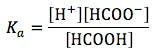Let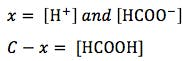(where C = initial concentration)

The expression for Ka can, therefore, be written as follows: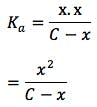Solving for x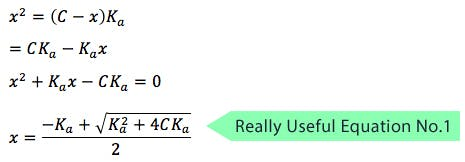x represents the hydrogen ion concentration [H+], and the equation is incredibly useful for calculating the pH of acidic buffer solutions.  You can substitute Kafor Kb and calculate the pH of basic solutions also.

Let’s work this equation through and calculate the pH of the two TFA solutions above;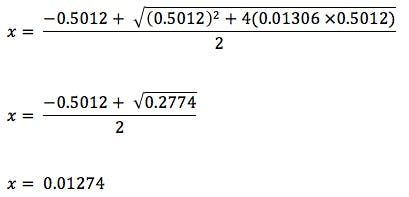Therefore pH =

pH = -log10 [H+]

pH = -log10 0.01274

pH = 1.89

You can work it out for yourself, but you will see that for the 0.1% w/v solution (molarity of 0.00877 g/mol-1), the pH turns out to be 2.06, a difference of 0.17 pH units.

Now, I can think of separations where this would make absolutely no difference whatsoever in the resolution between analytes, but I can also think of several, less-robust separations, which would be ruined if the eluent pH changed by this magnitude.

The art is recognising that there are different ways of making up 0.1% TFA, being sure you stipulate the method of preparation so others can reproduce your work, and designing separations which are robust enough to withstand small changes in pH.  Of course – my client was correct to say that the more the liquid density differs from unity (1), the bigger the difference in ultimate pH between % v/v and % w/v solutions.

There is another derivation of the Henderson-Hasselbalch equation which can also be very useful when making up buffer solutions for HPLC.

I’m often faced with the situation in which I need to make a buffer solution which will achieve a certain pH but am not sure what concentration of buffer to use.  This needn’t be difficult as you will see.

What concentration of buffer and acid are required to achieve a pH of 2.5 when using a buffer system of Formic Acid / Ammonium Formate with an overall concentration of 25mM (0.025M).

We can use the Henderson-Hasselbalch equation to calculate the required concentrations.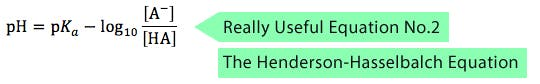[A-] represents the concentration of buffer and [HA] the concentration of acid.  The pKa of Formic acid is 3.77.

2.5 = 3.77 + Log10 [A-] / [HA]

Log10 [A-] / [HA] = 2.5 – 3.77 = -1.22

[A-] / [HA] = 10-1.22 = 0.06026

So we know
[A-] = 0.06026 [HA]

The total required concentration is 0.025 M
[A-] + [HA] = 0.025

So;
0.06026 [HA] + [HA] = 0.025
1.06026 [HA] = 0.025
[HA] = 0.025 / 1.06026 = 0.02358 M
[A-] = 0.06026 [HA]
[A-] = 0.06026 . 0.02358
[A-] = 1.42 X 10-3M

So, to make up 1 litre of ammonium formate buffer at pH 2.5 the solution concentrations need to be
0.02358 M Formic acid
0.00142 M Ammonium Formate

Many of us would have made up a solution of 0.25 M ammonium formate and used formic acid to adjust a stirred solution to pH 2.5, which is of course very simple and practical.  However by using the molecular weight of formic acid and ammonium formate we can very quickly calculate the mass of each reagent needed to make the eluent and improve the robustness of the procedure by preparing it gravimetrically.

Apologies for the rather heavy mathematical slant of the piece this time – however these equations are really useful in the lab and I hope that you will be able to use them both to improve the robustness of your separations, or at the very least be aware that % w/v and % v/v solutions can give different pH values depending upon the density of your reagents!